The Working of an atomizer depends upon

(a) Bernoulli's theorem                 (b) Boyle's law
(c) Archimedes principle               (d) Newton's law of motion

Concept Questions :-

Bernoulli theorem

Difficulty Level:

The pans of a physical balance are in equilibrium. Air is blown under the right hand pan; then the right hand pan will

(a) Move up

(b) Move down

(c) Move erratically

(d) Remain at the same level

Concept Questions :-

Bernoulli theorem
High Yielding Test Series + Question Bank - NEET 2020

Difficulty Level:

According to Bernoulli's equation

$\frac{\mathrm{P}}{\mathrm{\rho g}}+\mathrm{h}+\frac{1}{2}\frac{{\mathrm{v}}^{2}}{\mathrm{g}}=\mathrm{constant}$

The terms A, B and C are generally called respectively:

4. Gravity, pressure and velocity head

Concept Questions :-

Bernoulli theorem

Difficulty Level:

A sniper fires a rifle bullet into a gasoline tank making a hole 53.0 m below the surface of gasoline. The tank was sealed at 3.10 atm. The stored gasoline has a density of 660 ${\mathrm{kgm}}^{-3}$. The velocity with which gasoline begins to shoot out of the hole is
(a) 27.8 ${\mathrm{ms}}^{-1}$                                            (b) 41.0 ${\mathrm{ms}}^{-1}$
(c)  9.6 ${\mathrm{ms}}^{-1}$                                             (d) 19.7 ${\mathrm{ms}}^{-1}$

Concept Questions :-

Bernoulli theorem
High Yielding Test Series + Question Bank - NEET 2020

Difficulty Level:

An L-shaped tube with a small orifice is held in a water stream as shown in fig. The upper end of the tube is 10.6 cm above the surface of water. What will be the height of the jet of water coming from the orifice? Velocity of water stream is 2.45 m/s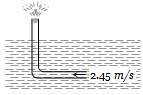(a) Zero                                         (b) 20.0 cm
(c) 10.6 cm                                    (d) 40.0 cm

Concept Questions :-

Bernoulli theorem
High Yielding Test Series + Question Bank - NEET 2020

Difficulty Level:

Fig. represents vertical sections of four wings moving horizontally in air. In which case the force is upwards

(a)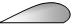(b)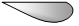(c)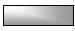(d)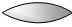Concept Questions :-

Bernoulli theorem

Difficulty Level:

A tank is filled with water up to a height H. Water is allowed to come out of a hole P in one of the walls at a depth D below the surface of water. Express the horizontal distance x in terms of H and D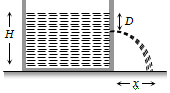(a) $\mathrm{x}=\sqrt{\mathrm{D}\left(\mathrm{H}-\mathrm{D}\right)}$

(b) $\mathrm{x}=\sqrt{\frac{\mathrm{D}\left(\mathrm{H}-\mathrm{D}\right)}{2}}$

(c) $\mathrm{x}=2\sqrt{\mathrm{D}\left(\mathrm{H}-\mathrm{D}\right)}$

(d) $\mathrm{x}=4\sqrt{\mathrm{D}\left(\mathrm{H}-\mathrm{D}\right)}$

Concept Questions :-

Bernoulli theorem
High Yielding Test Series + Question Bank - NEET 2020

Difficulty Level:

A rectangular vessel when full of water takes 10 minutes to be emptied through an orifice in its bottom. How much time will it take to be emptied when half filled with water
(a) 9 minute                        (b) 7 minute
(c) 5 minute                        (d) 3 minute

Concept Questions :-

Bernoulli theorem
High Yielding Test Series + Question Bank - NEET 2020

Difficulty Level:

A streamlined body falls through air from a height h on the surface of a liquid. If d and D(D > d) represents the densities of the material of the body and liquid respectively, then the time after which the body will be instantaneously at rest, is

(a) $\sqrt{\frac{2\mathrm{h}}{\mathrm{g}}}$                          (b) $\sqrt{\frac{2\mathrm{h}}{\mathrm{g}}·\frac{D}{d}}$

(c) $\sqrt{\frac{2\mathrm{h}}{\mathrm{g}}·\frac{d}{D}}$                    (d) $\sqrt{\frac{2\mathrm{h}}{\mathrm{g}}}\left(\frac{d}{D-d}\right)$

Concept Questions :-

Archimedes principle
High Yielding Test Series + Question Bank - NEET 2020

Difficulty Level:

A large tank of cross-section area A is filled with water to a height H. A small hole of area 'a' is made at the base of the tank. It takes time ${\mathrm{T}}_{1}$ to decrease the height of water to $\frac{\mathrm{H}}{\mathrm{\eta }}\left(\mathrm{\eta }>1\right)$ ; and it takes ${\mathrm{T}}_{2}$ time to take out the rest of water. If ${\mathrm{T}}_{1}={\mathrm{T}}_{2}$, then the value of $\mathrm{\eta }$ is
(a) 2                                    (b) 3
(c) 4                                    (d) $2\sqrt{2}$

Concept Questions :-

Bernoulli theorem